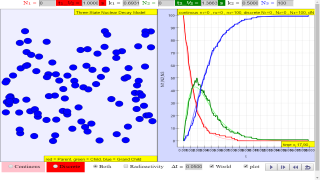Three-State Nuclear Decay

### Translations

Code Language Translator Run
es es es### Software Requirements

SoftwareRequirements

 Android iOS Windows MacOS with best with Chrome Chrome Chrome Chrome support full-screen? Yes. Chrome/Opera No. Firefox/ Samsung Internet Not yet Yes Yes cannot work on some mobile browser that don't understand JavaScript such as..... cannot work on Internet Explorer 9 and below

### CreditsWolfgang Christian - Davidson College; lookang

### Three State Decay Theory

A three-state radioactive sample. Radionuclides change from red to green to blue as they decay. If the number of nuclides is large, the upper state N1, intermediate state N2, and final state N3 populations obey the following coupled ordinary differential equations (ODEs)

$$\frac{dN_{1}}{dt} = - \lambda_{1}N_{1}$$

$$\frac{dN_{2}}{dt} = + \lambda_{1}N_{1} - \lambda_{2}N_{2}$$

$$\frac{dN_{3}}{dt} = - \lambda_{2}N_{2}$$

where λ1 is the upper state decay rate and λ2 is the intermediate state decay rate. The nature of the decay is governed by these decay constants. The Three State Nuclear Decay Model displays both the continuous ODE solution for these nuclide populations as well as a stochastic (probabilistic) solution that assumes integer nuclide populations and uses probability to determine if a radionuclide survives for a time Δt without decaying.

### Discrete decay model

The differential equation model produces continuous decay curves but hides the random and discrete nature of the underlying processes. A stochastic model that "rolls the dice" to determine if a radionuclide decays shows that the continuous model is only an approximation. The simulation computes the probably of decay between states 1->2, 2->3, and 1->3 during the finite time interval Δt. When the simulation is run, these probabilities are applied to each radioactive nucleus to determine if it decays. Note that there is a finite probability that a nuclide decays directly from state 1 to the stable state 3 within the time interval . Nature does not, however, skip state 2. We merely waited too long and the nuclide finished its decay before it was observed in state 2.

### Introduction

The Three State Nuclear Decay JavaScript Model extends the Two State Nuclear Decay JavaScript Model to simulate the radioactive decay of atomic nuclei in which the parent nucleus first decays into an intermediate state before decaying into a stable state. Although the decay of both the parent and intermediate nucleus (radionuclides) is spontaneous and unpredictable, the probability of decay of each radionuclide is constant and is usually known. The model displays a color-coded sample with N1 parent nuclides, N2 intermediate state nuclides, and N3 stable state nuclides. Before the simulation starts, users can set the initial numbers N1 and N2, the decay constants k1 and k2, and the time interval between measurements Δt. The simulation counts the number of decay events ΔN1 and ΔN2 within Δt and stops when all nuclides are in the stable state.

The plot shows the time evolution of the nuclides as well as the number of decay events in each Δt time interval to allow users to compare the data generated by the random decay model with a differential equation-based model as described on the Theory page. The Radioactivity check box switches the view to show the number of decay events ΔN within the Δt interval as described on the Theory page. The Particles check box enlarges the nuclide view.

### Credits:

The Three State Nuclear Decay model was developed by Wolfgang Christian using version 5.2 of the Easy Java/JavaScript Simulations (EjsS) authoring and modeling tool. Although EjsS is a Java program, it creates ready-to-run JavaScript simulations and ePubs. Information about EJS is available at: < http://www.um.es/fem/Ejs/> and in the OSP ComPADRE collection < http://www.compadre.org/OSP/>.

### Version:

1. http://weelookang.blogspot.sg/2011/01/ejs-open-source-decay-parent-daughter-g.html Blogpost remixing from Java to JavaScript by Loo Kang Wee
2. http://www.compadre.org/osp/items/detail.cfm?ID=13991 by JavaScript Version by Wolfgang Christian
3. http://www.compadre.org/osp/items/detail.cfm?ID=10577 by Java Version by Wolfgang Christian

[text]

### end faq